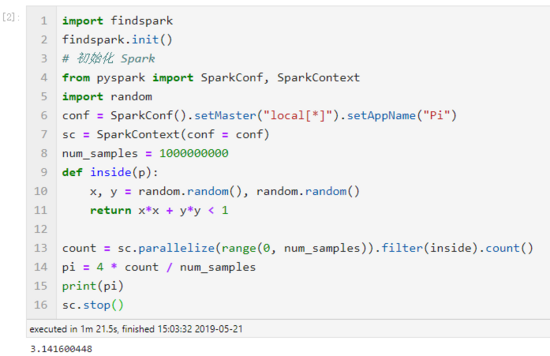Linux下搭建Spark 的 Python 编程环境的方法

Spark编程环境

Spark 可以独立安装使用，也可以和Hadoop 一起安装使用。在安装 Spark 之前，首先确保你的电脑上已经安装了 Java 8 或者更高的版本。

Spark 安装

```tar -xzf spark-2.4.2-bin-hadoop2.7.tgz

```# 编辑 zshrc 文件
sudo gedit ~/.zshrc
# 增加以下内容：export SPARK_HOME=/opt/spark-2.4.2export PATH=\$SPARK_HOME/bin:\$PATH
export <a href="https://www.linuxidc.com/topicnews.aspx?tid=17" target="_blank" title="Python">Python</a>PATH=\$SPARK_HOME/python:\$SPARK_HOME/python/lib/py4j-0.10.4-src.zip:\$PYTHONPATH```

PySpark in Jupyter

pyspark 将自动打开一个 Jupyter lab；
findSpark 包来加载 PySpark。

```export PYSPARK_DRIVER_PYTHON=jupyter
export PYSPARK_DRIVER_PYTHON_OPTS='lab'```

pysparkfindspark 包不是特定于 Jupyter lab 的，您也可以其它的 IDE 中使用该方法，因此这种方法更通用，也更推荐该方法。

`pip install findspark`

```# 导入 findspark 并初始化import findspark
findspark.init()from pyspark importSparkConf,SparkContextimport random
# 配置 Spark
conf =SparkConf().setMaster("local[*]").setAppName("Pi")# 利用上下文启动 Spark
sc =SparkContext(conf=conf)
num_samples =100000000definside(p):
x, y = random.random(), random.random()return x*x + y*y <1
count = sc.parallelize(range(0, num_samples)).filter(inside).count()
pi =4* count / num_samples
print(pi)
sc.stop()```PySpark in VScode

Visual Studio Code 作为一个优秀的编辑器，对于 Python 开发十分便利。这里首先推荐个人常用的一些插件：

Python：必装的插件，提供了Python语言支持；

Code Runner：支持运行文件中的某些片段；

```from pyspark importSparkContext,SparkConf
conf =SparkConf().setMaster("local[*]").setAppName("test")
sc =SparkContext(conf=conf)
logData = sc.textFile(logFile,2).cache()
numAs = logData.filter(lambda line:'a'in line).count()
numBs = logData.filter(lambda line:'b'in line).count()print("Lines with a: {0}, Lines with b:{1```